Study Materials: ncert solutions

Our ncert solutions for Exercise 4.3 Class 9 maths 4. Linear Equation In Two Variables - ncert solutions - Toppers Study is the best material for English Medium students cbse board and other state boards students.

Solutions ⇒ Class 9th ⇒ Mathematics ⇒ 4. Linear Equation In Two Variables

# Exercise 4.3 Class 9 maths 4. Linear Equation In Two Variables - ncert solutions - Toppers Study

Topper Study classes prepares ncert solutions on practical base problems and comes out with the best result that helps the students and teachers as well as tutors and so many ecademic coaching classes that they need in practical life. Our ncert solutions for Exercise 4.3 Class 9 maths 4. Linear Equation In Two Variables - ncert solutions - Toppers Study is the best material for English Medium students cbse board and other state boards students.

## Exercise 4.3 Class 9 maths 4. Linear Equation In Two Variables - ncert solutions - Toppers Study

CBSE board students who preparing for class 9 ncert solutions maths and Mathematics solved exercise chapter 4. Linear Equation In Two Variables available and this helps in upcoming exams 2023-2024.

### You can Find Mathematics solution Class 9 Chapter 4. Linear Equation In Two Variables

• All Chapter review quick revision notes for chapter 4. Linear Equation In Two Variables Class 9
• NCERT Solutions And Textual questions Answers Class 9 Mathematics
• Extra NCERT Book questions Answers Class 9 Mathematics
• Importatnt key points with additional Assignment and questions bank solved.

NCERT Solutions do not only help you to cover your syllabus but also will give to textual support in exams 2023-2024 to complete exercise 4.3 maths class 9 chapter 4 in english medium. So revise and practice these all cbse study materials like class 9 maths chapter 4.3 in english ncert book. Also ensure to repractice all syllabus within time or before board exams for ncert class 9 maths ex 4.3 in english.

See all solutions for class 9 maths chapter 4 exercise 4 in english medium solved questions with answers.

### Exercise 4.3 class 9 Mathematics Chapter 4. Linear Equation In Two Variables

• Exercise 4.3 Class 9 Maths 4. Linear Equation In Two Variables - Ncert Solutions - Toppers Study
• Class 9 Ncert Solutions
• Solution Chapter 4. Linear Equation In Two Variables Class 9
• Solutions Class 9
• Chapter 4. Linear Equation In Two Variables Exercise 4.3 Class 9

## Exercise 4.3 Class 9 maths 4. Linear Equation In Two Variables - ncert solutions - Toppers Study

Linear equation in two variable

Excecise : 4.3

1. Draw the graph of each of the following linear equations in two variables:

(i) x + y = 4

(ii) x y = 2

(iii) y = 3x

(iv) 3 = 2x + y

Solution :

(i) x+y = 4   =   y = 4-x(ii) x - y = 2    =  x = 2 + y(iii) y = 3x  = -3x + y    = y = 3x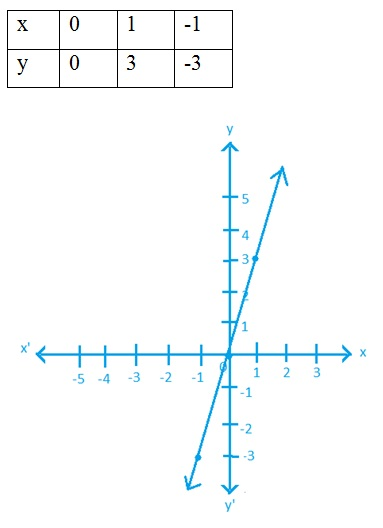2. Give the equations of two lines passing through (2, 14). How many more such lines are there, and why?

Solution :   x + y= 16

x - y=  -12

so, infinitely many lines can pass through these lines.

3. If the point (3, 4) lies on the graph of the equation 3y = ax + 7, find the value of a.

Solution :   3y = ax + 7

Putting the value of x and y

3(4) = a(3) +7    =   12 = 3a + 7    =    12 - 7 = 3a4. The taxi fare in a city is as follows: For the first kilo metre, the fare is ` 8 and for the subsequent distance it is ` 5 per km. Taking the distance covered as x km and total fare as Rs y, write a linear equation for this information, and draw its graph.

Solution :  distance covered = x

Total  fare           = y

Then, According To Question

8 + 5(x - 1) = y

= 8 + 5x - 5 = y

= 3 + 5x = y

= 5x -y + 3 = 0

= y = 5x + 3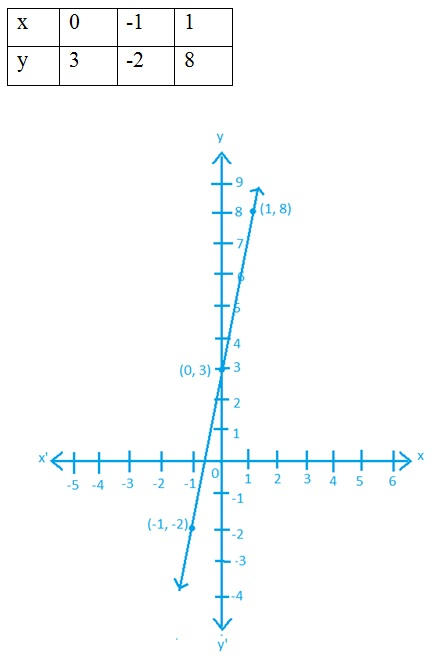5. From the choices given below, choose the equation whose graphs are given in Fig. 4.6 and Fig. 4.7.

For Fig. 4. 6                                 For Fig. 4.7

(i) y = x                                          (i) y = x + 2

(ii) x + y = 0                                  (ii) y = x – 2

(iii) y = 2x                                   (iii) y = –x + 2

(iv) 2 + 3y = 7x                          (iv) x + 2y = 6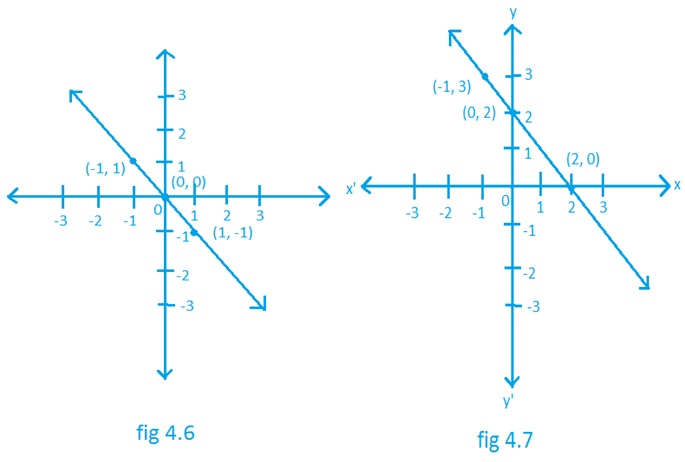Solution :  for fig. 4.6              for fig. 4.7

(ii) x + y = 0              (iii) y = -x + 2

6. If the work done by a body on application of a constant force is directly proportional to the distance travelled by the body, express this in the form of an equation in two variables and draw the graph of the same by taking the constant force as 5 units. Also read from the graph the work done when the distance travelled by the body is :

(i) 2 units                         (ii) 0 unit

Solution :   W= F×S

Constant force= 5 unit

Let the work done be y

Let the distance travelled be x

So,   y = 5x

(i) 2 units

Putting, x = 2

=   y = 5(2)

=   y =  10

(ii) 0 units

Putting, x= 0

=   y = 5(0)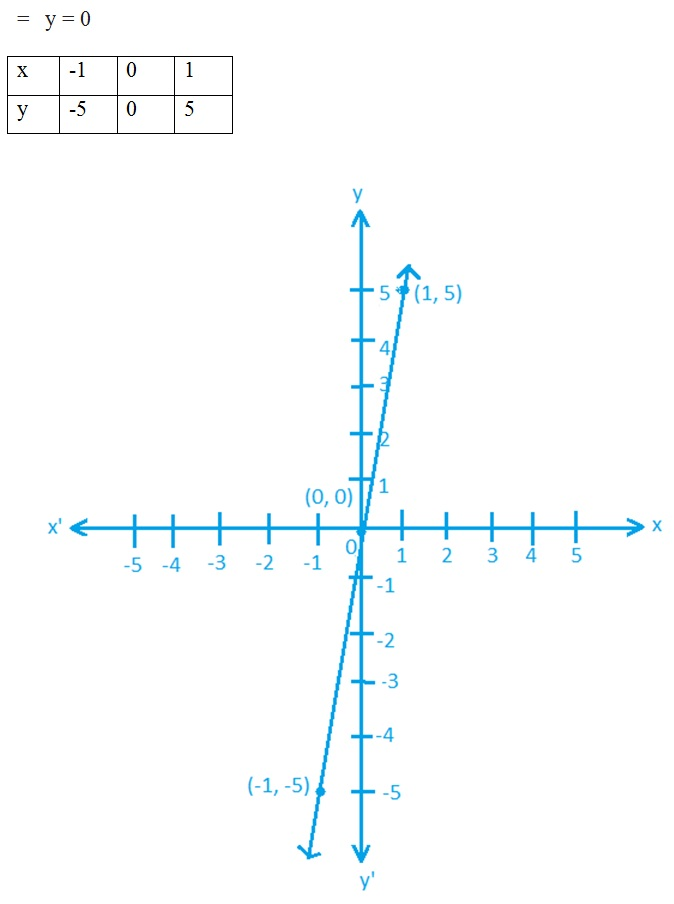7. Yamini and Fatima, two students of Class IX of a school, together contributed 100 towards the Prime Minister’s Relief  Fund to help the earthquake victims. Write a linear equation which satisfies this data. (You may take their contributions as ` x and ` y.) Draw the graph of the same.

Solution :

Together contributed = 100

Contribution by yamini = x

Contribution by fatima= y

According To Question,

x + y= 10

y = 100 - x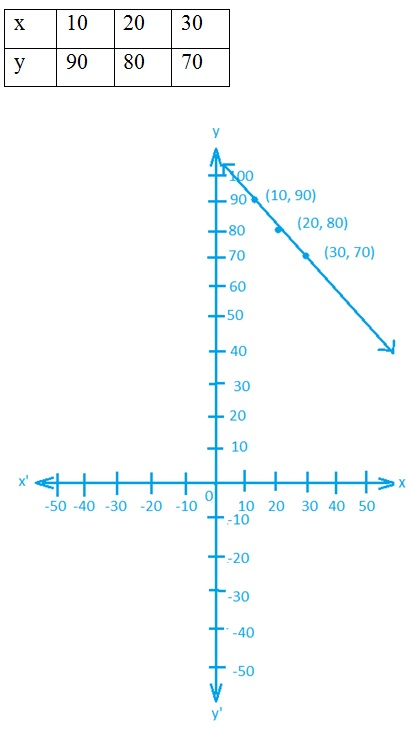8. In countries like USA and Canada, temperature is measured in Fahrenheit, whereas in countries like India, it is measured in Celsius. Here is a linear equation that converts Fahrenheit to Celsius: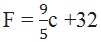(i) Draw the graph of the linear equation above using Celsius for x-axis and Fahrenheit for y-axis.

(ii) If the temperature is 30°C, what is the temperature in Fahrenheit?

(iii) If the temperature is 95°F, what is the temperature in Celsius?

(iv) If the temperature is 0°C, what is the temperature in Fahrenheit and if the temperature is 0°F, what is the temperature in Celsius?

(v) Is there a temperature which is numerically the same in both Fahrenheit and Celsius? If yes, find it.

Solution :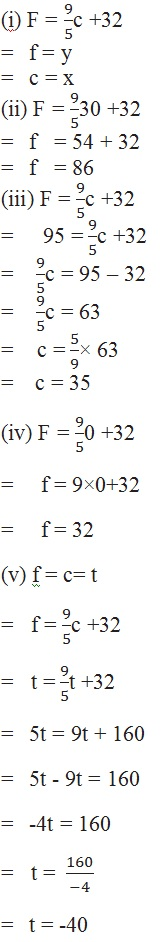##### Other Pages of this Chapter: 4. Linear Equation In Two Variables

Important Study materials for classes 06, 07, 08,09,10, 11 and 12. Like CBSE Notes, Notes for Science, Notes for maths, Notes for Social Science, Notes for Accountancy, Notes for Economics, Notes for political Science, Noes for History, Notes For Bussiness Study, Physical Educations, Sample Papers, Test Papers, Mock Test Papers, Support Materials and Books.Mathematics Class - 11th

NCERT Maths book for CBSE Students.

books

## Study Materials List:

##### Solutions ⇒ Class 9th ⇒ Mathematics
1. Number Systems
2. Polynomials
3. Coordinate Geometry
4. Linear Equation In Two Variables
5. Introduction To Euclid’s Geometry
6. Lines and Angles
7. Triangles